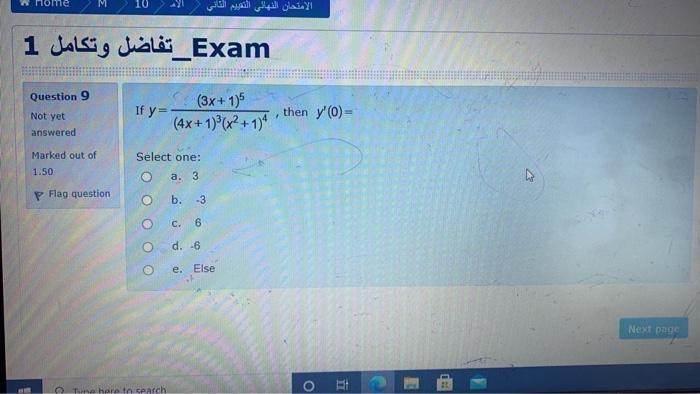### Create an Account

Home / Questions / الامتحان الأهلي التقييم الثاني ! Exam_تفاضل وتكامل 1 Question 9 If y= (3x+1) (4x + 1)(x2+1...

# الامتحان الأهلي التقييم الثاني ! Exam_تفاضل وتكامل 1 Question 9 If y= (3x+1) (4x + 1)(x2+1) Not yet answered then y'O- Marked out of 1.50 Select one: a. 3 m. Flag question o b. -3 c. 6 d. -6 e. Else N

الامتحان الأهلي التقييم الثاني ! Exam_تفاضل وتكامل 1 Question 9 If y= (3x+1) (4x + 1)(x2+1) Not yet answered then y'O- Marked out of 1.50 Select one: a. 3 m. Flag question o b. -3 c. 6 d. -6 e. Else Next page o i E E Tune here to searchMay 09 2021 View more View LessSubscribe To Get Solution